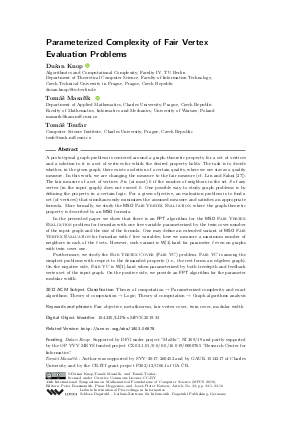Document# Parameterized Complexity of Fair Vertex Evaluation Problems

### Authors Dušan Knop, Tomáš Masařík, Tomáš Toufar## File

LIPIcs.MFCS.2019.33.pdf
• Filesize: 0.56 MB
• 16 pages

## Cite As

Dušan Knop, Tomáš Masařík, and Tomáš Toufar. Parameterized Complexity of Fair Vertex Evaluation Problems. In 44th International Symposium on Mathematical Foundations of Computer Science (MFCS 2019). Leibniz International Proceedings in Informatics (LIPIcs), Volume 138, pp. 33:1-33:16, Schloss Dagstuhl - Leibniz-Zentrum für Informatik (2019)
https://doi.org/10.4230/LIPIcs.MFCS.2019.33

## Abstract

A prototypical graph problem is centered around a graph-theoretic property for a set of vertices and a solution to it is a set of vertices for which the desired property holds. The task is to decide whether, in the given graph, there exists a solution of a certain quality, where we use size as a quality measure. In this work, we are changing the measure to the fair measure (cf. Lin and Sahni [Li-Shin Lin and Sartaj Sahni, 1989]). The fair measure of a set of vertices S is (at most) k if the number of neighbors in the set S of any vertex (in the input graph) does not exceed k. One possible way to study graph problems is by defining the property in a certain logic. For a given objective, an evaluation problem is to find a set (of vertices) that simultaneously minimizes the assumed measure and satisfies an appropriate formula. More formally, we study the {MSO} Fair Vertex Evaluation, where the graph-theoretic property is described by an {MSO} formula. In the presented paper we show that there is an FPT algorithm for the {MSO} Fair Vertex Evaluation problem for formulas with one free variable parameterized by the twin cover number of the input graph and the size of the formula. One may define an extended variant of {MSO} Fair Vertex Evaluation for formulas with l free variables; here we measure a maximum number of neighbors in each of the l sets. However, such variant is {W}-hard for parameter l even on graphs with twin cover one. Furthermore, we study the Fair Vertex Cover (Fair VC) problem. Fair VC is among the simplest problems with respect to the demanded property (i.e., the rest forms an edgeless graph). On the negative side, Fair VC is {W}-hard when parameterized by both treedepth and feedback vertex set of the input graph. On the positive side, we provide an FPT algorithm for the parameter modular width.

## Subject Classification

##### ACM Subject Classification
• Theory of computation → Parameterized complexity and exact algorithms
• Theory of computation → Logic
• Theory of computation → Graph algorithms analysis
##### Keywords
• Fair objective
• metatheorem
• fair vertex cover
• twin cover
• modular width

## Metrics

• Access Statistics
• Total Accesses (updated on a weekly basis)
0